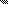# MINLPLib

### A Library of Mixed-Integer and Continuous Nonlinear Programming Instances

#### Instance: ex6_1_1

 Formatsⓘ ams gms mod nl osil Primal Bounds (infeas ≤ 1e-08)ⓘ -0.02019831 p1 ( gdx sol ) (infeas: 9e-18) Other points (infeas > 1e-08)ⓘ Dual Boundsⓘ -0.02019924 (ANTIGONE)-0.02020129 (BARON)-0.02019831 (COUENNE)-0.02019912 (LINDO)-0.02020416 (SCIP) Referencesⓘ Floudas, C A, Pardalos, Panos M, Adjiman, C S, Esposito, W R, Gumus, Zeynep H, Harding, S T, Klepeis, John L, Meyer, Clifford A, and Schweiger, C A, Handbook of Test Problems in Local and Global Optimization, Kluwer Academic Publishers, 1999.McDonald, C M and Floudas, C A, GLOPEQ: A New Computational Tool for the Phase and Chemical Equilibrium Problem, Computers and Chemical Engineering, 21:1, 1997, 1-23.Heidemann, R and Mandhane, J, Some Properties of the NRTL Equation in Correlating Liquid-Liquid Equilibrium Data, Chemical Engineering Science, 28:5, 1973, 1213-1221. Sourceⓘ Test Problem ex6.1.1 of Chapter 6 of Floudas e.a. handbook Added to libraryⓘ 31 Jul 2001 Problem typeⓘ NLP #Variablesⓘ 8 #Binary Variablesⓘ 0 #Integer Variablesⓘ 0 #Nonlinear Variablesⓘ 8 #Nonlinear Binary Variablesⓘ 0 #Nonlinear Integer Variablesⓘ 0 Objective Senseⓘ min Objective typeⓘ nonlinear Objective curvatureⓘ indefinite #Nonzeros in Objectiveⓘ 8 #Nonlinear Nonzeros in Objectiveⓘ 8 #Constraintsⓘ 6 #Linear Constraintsⓘ 2 #Quadratic Constraintsⓘ 4 #Polynomial Constraintsⓘ 0 #Signomial Constraintsⓘ 0 #General Nonlinear Constraintsⓘ 0 Operands in Gen. Nonlin. Functionsⓘ mul log Constraints curvatureⓘ indefinite #Nonzeros in Jacobianⓘ 16 #Nonlinear Nonzeros in Jacobianⓘ 12 #Nonzeros in (Upper-Left) Hessian of Lagrangianⓘ 24 #Nonzeros in Diagonal of Hessian of Lagrangianⓘ 4 #Blocks in Hessian of Lagrangianⓘ 2 Minimal blocksize in Hessian of Lagrangianⓘ 4 Maximal blocksize in Hessian of Lagrangianⓘ 4 Average blocksize in Hessian of Lagrangianⓘ 4.0 #Semicontinuitiesⓘ 0 #Nonlinear Semicontinuitiesⓘ 0 #SOS type 1ⓘ 0 #SOS type 2ⓘ 0 Infeasibility of initial pointⓘ 1.11e-16 Sparsity JacobianⓘSparsity Hessian of Lagrangianⓘ```\$offlisting
*
*  Equation counts
*      Total        E        G        L        N        X        C        B
*          7        7        0        0        0        0        0        0
*
*  Variable counts
*                   x        b        i      s1s      s2s       sc       si
*      Total     cont   binary  integer     sos1     sos2    scont     sint
*          9        9        0        0        0        0        0        0
*  FX      0
*
*  Nonzero counts
*      Total    const       NL      DLL
*         25        5       20        0
*
*  Solve m using NLP minimizing objvar;

Variables  objvar,x2,x3,x4,x5,x6,x7,x8,x9;

Positive Variables  x6,x7,x8,x9;

Equations  e1,e2,e3,e4,e5,e6,e7;

e1.. -((log(x2) - log(x2 + x4))*x2 + (log(x4) - log(x2 + x4))*x4 + (log(x3) -
log(x3 + x5))*x3 + (log(x5) - log(x3 + x5))*x5 + 0.925356626778358*x2*x8
+ 0.746014540096753*x4*x6 + 0.925356626778358*x3*x9 + 0.746014540096753*
x5*x7) + objvar =E= 0;

e2.. x6*(x2 + 0.159040857374844*x4) - x2 =E= 0;

e3.. x7*(x3 + 0.159040857374844*x5) - x3 =E= 0;

e4.. x8*(0.307941026821595*x2 + x4) - x4 =E= 0;

e5.. x9*(0.307941026821595*x3 + x5) - x5 =E= 0;

e6..    x2 + x3 =E= 0.5;

e7..    x4 + x5 =E= 0.5;

* set non-default bounds
x2.lo = 1E-7; x2.up = 0.5;
x3.lo = 1E-7; x3.up = 0.5;
x4.lo = 1E-7; x4.up = 0.5;
x5.lo = 1E-7; x5.up = 0.5;

* set non-default levels
x2.l = 0.4993;
x3.l = 0.0007;
x4.l = 0.3441;
x5.l = 0.1559;
x6.l = 0.901221308981222;
x7.l = 0.0274569351394739;
x8.l = 0.691165161172019;
x9.l = 0.998619236157215;

Model m / all /;

m.limrow=0; m.limcol=0;
m.tolproj=0.0;

\$if NOT '%gams.u1%' == '' \$include '%gams.u1%'

\$if not set NLP \$set NLP NLP
Solve m using %NLP% minimizing objvar;

```

Last updated: 2021-03-11 Git hash: 66559cbc